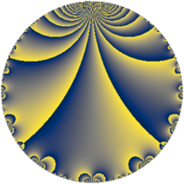# Properties

 Label 9.4.aLevel $9$ Weight $4$ Character orbit 9.a Rep. character $\chi_{9}(1,\cdot)$ Character field $\Q$ Dimension $1$ Newform subspaces $1$ Sturm bound $4$ Trace bound $0$

# Related objects

## Defining parameters

 Level: $$N$$ $$=$$ $$9 = 3^{2}$$ Weight: $$k$$ $$=$$ $$4$$ Character orbit: $$[\chi]$$ $$=$$ 9.a (trivial) Character field: $$\Q$$ Newform subspaces: $$1$$ Sturm bound: $$4$$ Trace bound: $$0$$

## Dimensions

The following table gives the dimensions of various subspaces of $$M_{4}(\Gamma_0(9))$$.

Total New Old
Modular forms 5 2 3
Cusp forms 1 1 0
Eisenstein series 4 1 3

The following table gives the dimensions of the cuspidal new subspaces with specified eigenvalues for the Atkin-Lehner operators and the Fricke involution.

$$3$$Dim.
$$+$$$$1$$

## Trace form

 $$q - 8q^{4} + 20q^{7} + O(q^{10})$$ $$q - 8q^{4} + 20q^{7} - 70q^{13} + 64q^{16} + 56q^{19} - 125q^{25} - 160q^{28} + 308q^{31} + 110q^{37} - 520q^{43} + 57q^{49} + 560q^{52} + 182q^{61} - 512q^{64} - 880q^{67} + 1190q^{73} - 448q^{76} + 884q^{79} - 1400q^{91} - 1330q^{97} + O(q^{100})$$

## Decomposition of $$S_{4}^{\mathrm{new}}(\Gamma_0(9))$$ into newform subspaces

Label Dim. $$A$$ Field CM Traces A-L signs $q$-expansion
$$a_2$$ $$a_3$$ $$a_5$$ $$a_7$$ 3
9.4.a.a $$1$$ $$0.531$$ $$\Q$$ $$\Q(\sqrt{-3})$$ $$0$$ $$0$$ $$0$$ $$20$$ $$+$$ $$q-8q^{4}+20q^{7}-70q^{13}+2^{6}q^{16}+\cdots$$